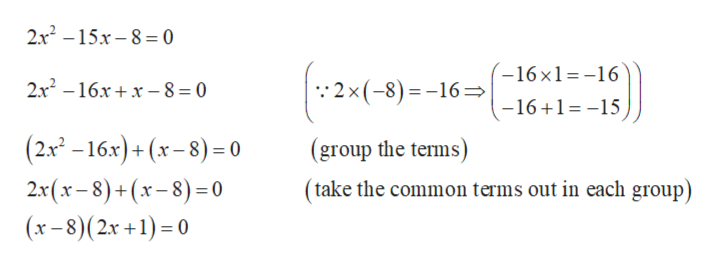2x2 - 15x - 8 = 0How do I solve this by Factoring?

Question

2x- 15x - 8 = 0

How do I solve this by Factoring?

Step 1

Factor the equation...help_outlineImage Transcriptionclose2x2 -15x8 0 (-16 x1 =-16 2x (-8) 16 2x2 -16xx8 = 0 -16+1=-15 (2x2 -16x)+(x-8)= 0 2x(x-8)+(x-8)0 (x-8)(2x +1)0 (group the terms) (take the common terms out in each group) fullscreen

Want to see the full answer?

See Solution

Want to see this answer and more?

Our solutions are written by experts, many with advanced degrees, and available 24/7

See Solution
Tagged in

Algebra